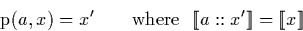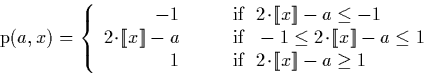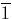Next: Dyadic Stream Operations Up: Auxiliary operations Previous: Addition or subtraction of

## The p' function

We now give another useful algorithm for a function which we will dub the p' function. It is used extensively in situations where you can perform an analysis to determine the range of the output of some operation using the method described in section 3.6.2, but do not know what the first digit of a resulting stream will be. It is used in a number of algorithms, including for example the division algorithm (section 4.4.4), and the algorithm used to compute the limits of Cauchy sequence described in chapter 5).

The p' function re-expresses a stream starting with an unknown digit as a stream which, when appended to a specified digit, represents the same number as the original stream. In other words, the p' function of the digit a and stream x works as follows:The caveat associated with using the p' function is that if it is impossible to re-express the stream starting with the specified digit, the result will be undefined. The p' function removing the digit a from the stream x may be defined as follows:The algorithm may be implemented simply by examining cases. Where the start digit of the stream is equal to the digit being removed, the result is simply the tail of the input stream. If the digit at the head of the stream differs by one from the digit to be removed, the functions to compute (x-1) and (x+1) defined in section 4.1.3 may be used. If the digits differ by two (ie. 1 and), the resulting stream will be either minus one (-1) or one (1). Hence:

 = x' p(1,0::x') = x' - 1 p(1,1::x') = -1 p(0,0::x') = x' p(0,1::x') = x' - 1 p(1,0::x') = 1 - x' p(1,1::x') = x'Next: Dyadic Stream Operations Up: Auxiliary operations Previous: Addition or subtraction of
Martin Escardo
5/11/2000